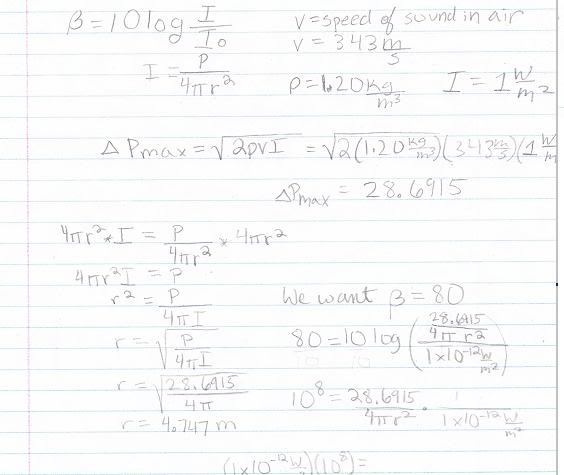# Given Sound-Level B1 at A Distance D1, Find D2 at Sound Level B2

I'm here to check my work again. I hope you don't mind. I'm going to try to check as many as I can today. :)

The sound-level 2.0 m from a pneumatic chipper is 120 dB. Assuming it radiates uniformly in all directions, how far from it must you be in order for the level to drop 40 dB down to something more comfortable?
Given:
D1 = 2.0 m
B1 = 120 dB
D2 = x
B2 = 80 dB
Find x. I decided I could use the inverse square law. As the distance increases, the energy decreases in proportion to the distance squared.

$$\frac{B1}{B2}$$ = $$\frac{D2^2}{D1^2}$$

$$\frac{120}{80}$$ = $$\frac{x^2}{4}$$
x= sqrt((120*4)/80) = 2.4495 m.

In order to hear 40 dB less, I need to walk 2.4495 m from my current distance. The total distance I stand from the chipper is 2 +2.4495 = 4.4495 m. Am I correct?

ideasrule
Homework Helper
B1/B2=D12/D22 only works if all four values are measured on a linear scale. Since the decibel scale is logarithmic, it doesn't work.

40 decibel is 4 bels, which corresponds to a factor of 10^4=10000. So the sound needs to be 10 000 times quieter. Now you can use that formula, but don't add your initial distance to D2. D2 is already the listener's distance from the sound source.

I'm sorry I don't understand how what you have told me allows me to use the inverse square law, so I will try it using the normal equations. However, thanks for pointing out that I do not have to add the D1 and D2. You say 104=10000 will allow me to use my inverse square law. I understand that you're saying that because 10 to the power of something relates to log. When you say 10log(something), it equals that something. However, I don't see how a conversion to bells in this manner helps. Thanks, though.

Using B = 10log(I/Io)

120dB = 10log(I/(1x10-12)
I = 1 W/m280 = 10 log(I/10-12) = 10-4 W/m2
120 = 10 log(I/10-12) = 1 W/m2

P = 4$$\pi$$*r2
P = 4$$\pi$$*22 = 50.27

r = $$\sqrt{\frac{P}{I*4*\pi}}$$
r = $$\sqrt{\frac{50.27}{I*4*\pi}}$$ = 200 m